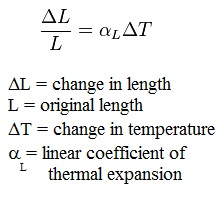> > Thermal Expansion

# Thermal Expansion

Have you ever noticed that small gap in the railway tracks? Why do you think they are there? Or, have you ever noticed your mom trying to open up a jammed bottle, by heating up near its neck? What is this magic all about? Let’s find out more about the process of thermal expansion here.

### Suggested VideosHeat and Effect of HeatIntroduction to Heat and TemperartureHeat is up## Thermal Expansion

If the temperature increases, then the volume of the material also increases. Generally, this is known as thermal expansion. We can express it in this way that it is the fractional change in length or volume per unit change in temperature. In case of expansion of a solid, normally linear expansion coefficient is usually employed.

In case of thermal expansion of solid, it is described in terms of change in length, height, and thickness. For liquid and gas, the volume expansion coefficient is more useful. Generally, if the material is a fluid then we can describe it in terms of change in volume.Among the atoms and molecules, the bonding forces vary from material to material. Characteristics of elements and compounds are known as expansion coefficient. If a crystalline solid has the same structural configuration throughout, (isometric) then in all dimensions of crystal the expansion will be uniform.

If the crystal is not isometric then expansion coefficient is also different for different crystallographic directions and as the temperature will change then the crystal will also change the shape. Softer materials have a higher coefficient of expansion (CTE) but harder materials like tungsten have lower CTE.

### Types of Expansion

• Linear Expansion: Linear expansion is defined as the increase in the length of the solid. Example: If we will consider one rod where the length of the rod is l, and we will increase the temperature of a rod by a small amount.  So Linear Expansion is given by:•  The Coefficient of linear expansion of the given solid is denoted as a. then for a unit is per degree Celsius) in the CGS and in the SI system it is per kelvin K-1.
• Volume Expansion: Volume expansion is defined as the increase in the volume of the solid on heating. With a change in temperature ∆t the change in volume of a solid is given by ∆v=Vy∆t where the coefficient of volume expansion is y.
• Area or superficial Expansion: Superficial expansion is defined as the increase in surface area of the solid on heating. If you consider at 00C area of a solid is A0 then its area at t0c is given by: A0(l+βt). Unit of β is 0C-1 or K-1. Where β is known as the coefficient of superficial expansion.

6α = 3β = 2γ

This equation shows the relationship between α is the linear expansion, β is the superficial expansion, is volume expansion. These three coefficients of expansion for a given solid are not constant because these values totally depend on the temperature. Examples of thermal expansion in our daily life are thermometers, riveting, on wooden wheels fixing metal tires etc.

## Solved Example For You

Q. In one continuous piece from a roll of a sheet of aluminum modern eavestroughs are constructed. For a 30-meter-long what is the change in length? Where α=23×10-6C-1 for temperature range ∆T=100F.

Ans: ∆L= L0 α ∆T

= (30m) (23× 10-6)(500/9) = 3.8 cm

Share with friends

## Customize your course in 30 seconds

##### Which class are you in?
5th
6th
7th
8th
9th
10th
11th
12th
Get ready for all-new Live Classes!
Now learn Live with India's best teachers. Join courses with the best schedule and enjoy fun and interactive classes.Ashhar Firdausi
IIT Roorkee
BiologyDr. Nazma Shaik
VTU
ChemistryGaurav Tiwari
APJAKTU
Physics
Get Started

## Browse

##### Thermal Properties of MatterSubscribe
Notify of

## Question Mark?

Have a doubt at 3 am? Our experts are available 24x7. Connect with a tutor instantly and get your concepts cleared in less than 3 steps.